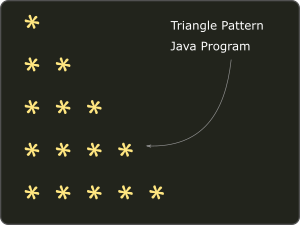Loops are used by every programmer whether he is a beginner or an expert. Like all other languages, java also has for-loops.

## Why use a loop?

Loops are used when we want to iterate over some statements multiple times based on some condition.

There are mainly four types of loops in Java and they are as below-

In this tutorial, we are going to learn and understand for-loop in Java.

## When to use for-loop in Java?

For-loop in java has wide uses.

The java for-loop is a control flow statement.
It helps to iterate over some statements until a given condition matches.

For-loops are recommended if the number of iteration is known or fixed.

Let us see the syntax of for-loop.

### Java for-loop Syntax

``````for(initialization ; condition ; increament/decreament)
{
//loop body
statement 1;
statement 2;
...
statement n;
}``````

First, let us get familiar with these terms.

1. initialization – We initialize the loop variable here or use some pre-initialized variable. This is the loop variable. This statement executes only once when the loop starts.
2. condition –  The condition statement is checked every time the loop executes. If the condition statement is false control does not enter into the loop.
3. increment/decrement- This statement is checked every time except the very first time the loop executes. Moreover, the statement is used to increase or decrease the loop variable.

For your better understanding, a diagram is provided below.Java for-loop working flow diagram

Finally. Let’s see how to code a for loop in Java.
Above all, coding is very important.

### Example: Find a multiplication table for a given number using Java for loop

``````
import java.util.Scanner;
public class ForLoopExample {

public static void main(String[] args) {
Scanner sc=new Scanner(System.in);
System.out.println("Enter a number whose multiplication table you want");
int num=sc.nextInt();
int table=0;
System.out.println("Multiplication table for "+ num);
for(int i=1;i<=10;i++)
{
table=num*i;
System.out.println(num+" * "+i+ " = " +table);
}
}

}
``````

Output

``````Enter a number whose multiplication table you want
8
Multiplication table for 8
8 * 1 = 8
8 * 2 = 16
8 * 3 = 24
8 * 4 = 32
8 * 5 = 40
8 * 6 = 48
8 * 7 = 56
8 * 8 = 64
8 * 9 = 72
8 * 10 = 80
``````

## Nested for-loop in Java

For loop inside a for loop is called nested for-loop.
The inner for loop execute if and only if control enters into the outer for loop.

It sounds a bit confusing so let’s understand it with an example.

### Example: Print the following  triangle star pattern using For Loop*pattern Java code

To print the triangle pattern we need a nested for loop, one loop(outer loop) for row and another(inner loop) for the column.

``````
public class NestedForLoop {

public static void main(String[] args) {
for(int i=1;i<=5;i++)
{
for(int j=1;j<=i;j++)
{
System.out.print("* ");
}
System.out.println();
}

}

}

``````Output

## Be careful with Infinite for-loop

A loop becomes infinite when its condition is always true. For-loops can be infinite too. Therefore it does not terminate.

Even though in most of the cases we avoid creating infinite loops but there could be some cases where we need to create it.
In such cases, the loop will terminate when the application exits.

Or if by mistake you land into infinite for loop then use ` ctrl+c` in terminal to terminate it. In eclipse, we use the red dot in the top right corner of the console to terminate the infinite loop.

Example of infinite for-loop

``````public class InfiniteForLoop {

public static void main(String[] args) {
for(;;)
{
System.out.println("Letstacle");
}

}

}

``````

Or something like this

``````
for (int i = 1; i < 10;) {
System.out.println("Letstacle");
}
``````

Output

``````Letstacle
Letstacle
Letstacle
Letstacle
Letstacle
Letstacle
Letstacle
Letstacle
Letstacle
...
``````

Here, the condition is always true because we have not updated the value of “i”. Thus “i” always remains 1 and the loop becomes infinite.Like this article? Follow us on Facebook and LinkedIn. You can also subscribe to our weekly Feed